Skip to content
Related Articles
Data Normalization with Pandas
• Last Updated : 11 Dec, 2020

In this article, we will learn how to normalize data in Pandas. Let’s discuss some concepts first :

• Pandas: Pandas is an open-source library that’s built on top of NumPy library. it is a Python package that provides various data structures and operations for manipulating numerical data and statistics. It’s mainly popular for importing and analyzing data much easier. Pandas is fast and it’s high-performance & productive for users.
• Data Normalization: Data Normalization could also be a typical practice in machine learning which consists of transforming numeric columns to a standard scale. In machine learning, some feature values differ from others multiple times. The features with higher values will dominate the learning process.

### Steps Needed

Here, we will apply some techniques to normalize the data and discuss these with the help of examples. For this, let’s understand the steps needed for data normalization with Pandas.

1. Import Library (Pandas)
2. Import / Load / Create data.
3. Use the technique to normalize the data.

### Examples

Here, we create data by some random values and apply some normalization techniques to it.

## Python3

 `# importing packages``import` `pandas as pd`` ` `# create data``df ``=` `pd.DataFrame([``                   ``[``180000``, ``110``, ``18.9``, ``1400``], ``                   ``[``360000``, ``905``, ``23.4``, ``1800``], ``                   ``[``230000``, ``230``, ``14.0``, ``1300``], ``                   ``[``60000``, ``450``, ``13.5``, ``1500``]], ``   ` `                   ``columns``=``[``'Col A'``, ``'Col B'``,``                            ``'Col C'``, ``'Col D'``])`` ` `# view data``display(df)`

Output: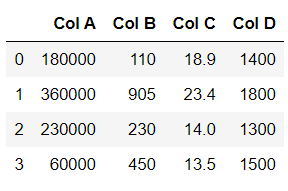See the plot of this dataframe:

## Python3

 `import` `matplotlib.pyplot as plt``df.plot(kind ``=` `'bar'``)`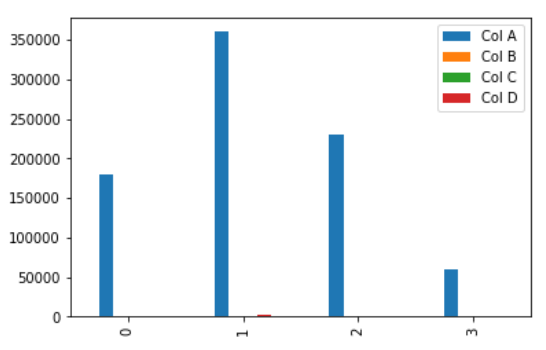### Using The maximum absolute scaling

The maximum absolute scaling rescales each feature between -1 and 1 by dividing every observation by its maximum absolute value. We can apply the maximum absolute scaling in Pandas using the .max() and .abs() methods, as shown below.

## Python3

 `# copy the data``df_max_scaled ``=` `df.copy()`` ` `# apply normalization techniques``for` `column ``in` `df_max_scaled.columns:``    ``df_max_scaled[column] ``=` `df_max_scaled[column]  ``/` `df_max_scaled[column].``abs``().``max``()``     ` `# view normalized data``display(df_max_scaled)`

Output :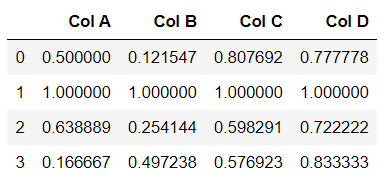See the plot of this dataframe:

## Python3

 `import` `matplotlib.pyplot as plt``df_max_scaled.plot(kind ``=` `'bar'``)`

Output: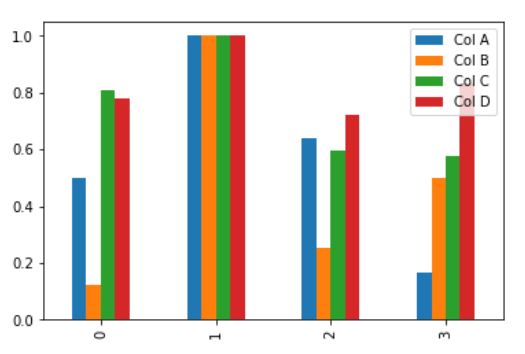### Using The min-max feature scaling

The min-max approach (often called normalization) rescales the feature to a hard and fast range of [0,1] by subtracting the minimum value of the feature then dividing by the range. We can apply the min-max scaling in Pandas using the .min() and .max() methods.

## Python3

 `# copy the data``df_min_max_scaled ``=` `df.copy()`` ` `# apply normalization techniques``for` `column ``in` `df_min_max_scaled.columns:``    ``df_min_max_scaled[column] ``=` `(df_min_max_scaled[column] ``-` `df_min_max_scaled[column].``min``()) ``/` `(df_min_max_scaled[column].``max``() ``-` `df_min_max_scaled[column].``min``())    `` ` `# view normalized data``print``(df_min_max_scaled)`

Output :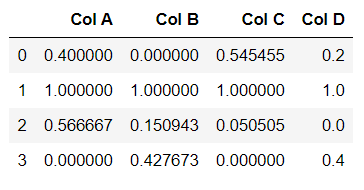Let’s draw a plot with this dataframe:

## Python3

 `import` `matplotlib.pyplot as plt``df_min_max_scaled.plot(kind ``=` `'bar'``)`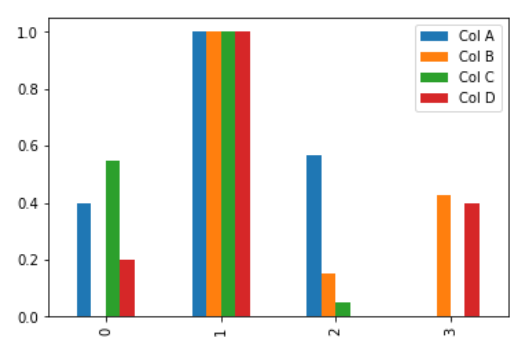### Using The z-score method

The z-score method (often called standardization) transforms the info into distribution with a mean of 0 and a typical deviation of 1. Each standardized value is computed by subtracting the mean of the corresponding feature then dividing by the quality deviation.

## Python3

 `# copy the data``df_z_scaled ``=` `df.copy()`` ` `# apply normalization techniques``for` `column ``in` `df_z_scaled.columns:``    ``df_z_scaled[column] ``=` `(df_z_scaled[column] ``-``                           ``df_z_scaled[column].mean()) ``/` `df_z_scaled[column].std()    `` ` `# view normalized data   ``display(df_z_scaled)`

Output :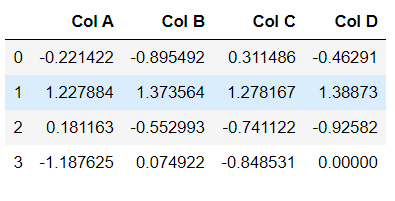Let’s draw a plot with this dataframe.

## Python3

 `import` `matplotlib.pyplot as plt``df_z_scaled.plot(kind``=``'bar'``)`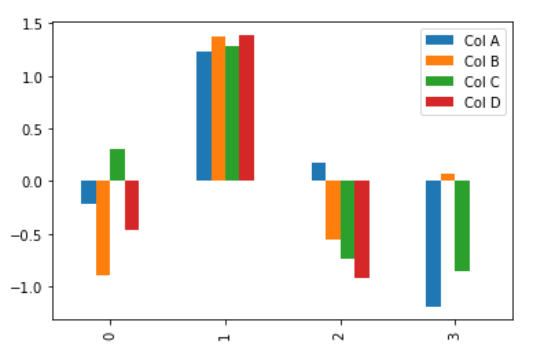### Summary

Data normalization consists of remodeling numeric columns to a standard scale. In Python, we will implement data normalization in a very simple way. The Pandas library contains multiple built-in methods for calculating the foremost common descriptive statistical functions which make data normalization techniques very easy to implement.

Attention geek! Strengthen your foundations with the Python Programming Foundation Course and learn the basics.

To begin with, your interview preparations Enhance your Data Structures concepts with the Python DS Course. And to begin with your Machine Learning Journey, join the Machine Learning – Basic Level Course

My Personal Notes arrow_drop_up